I’ve been playing with paper folding recently, and exploring the mathematics involved. I’m simply amazed by the number of mathematical ideas that can be represented by paper folding, so I thought I would share a few of my discoveries here.

Sequences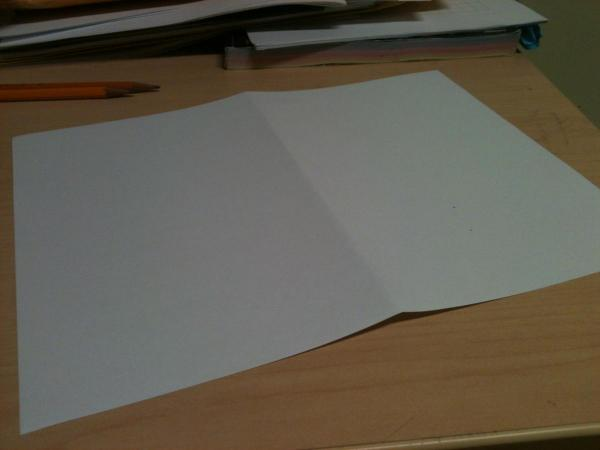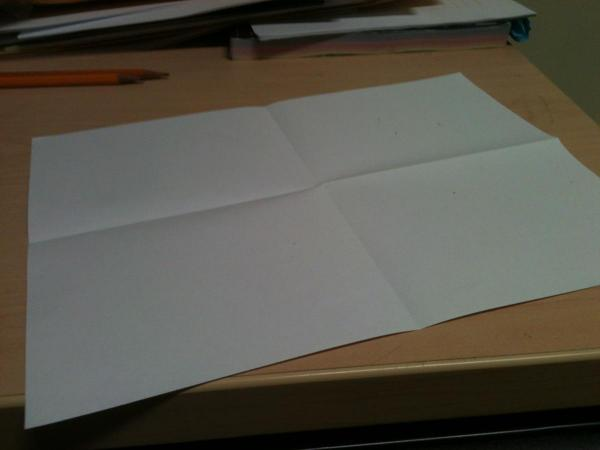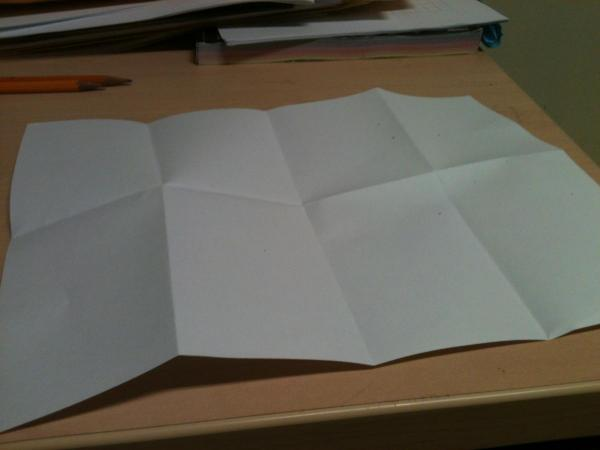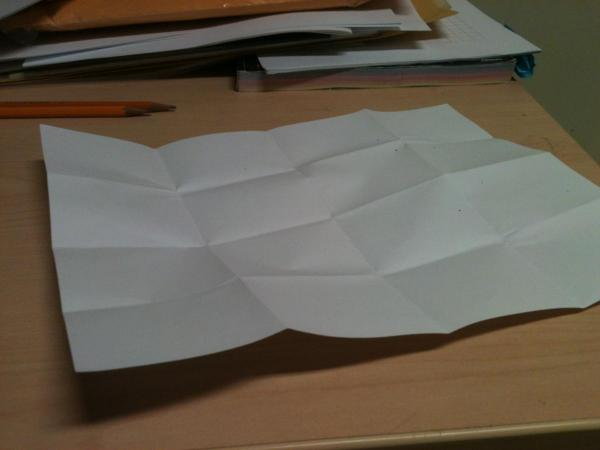As you can see above, you can generate the sequence of numbers 1, 2, 4, 8, 16, 32 and so on, just by folding the paper in half again each time. This means that there is an exponential relationship between the number of folds you have made and the number of areas created on the paper.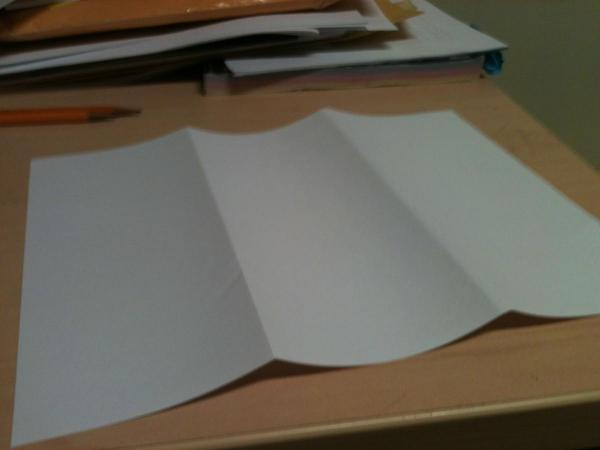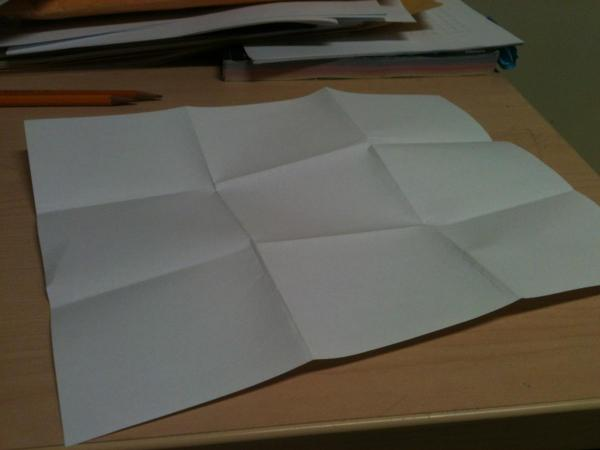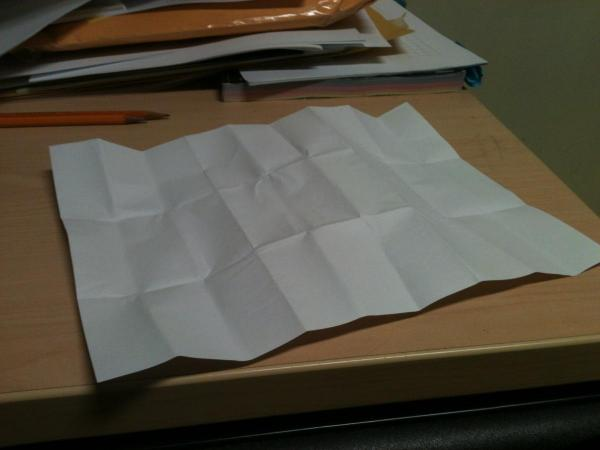Notice that if I instead fold the paper into thirds each time, the sequence changes into 1, 3, 9, 27, etc… which suggests that folding a piece of paper is a little bit like multiplication.

Fractions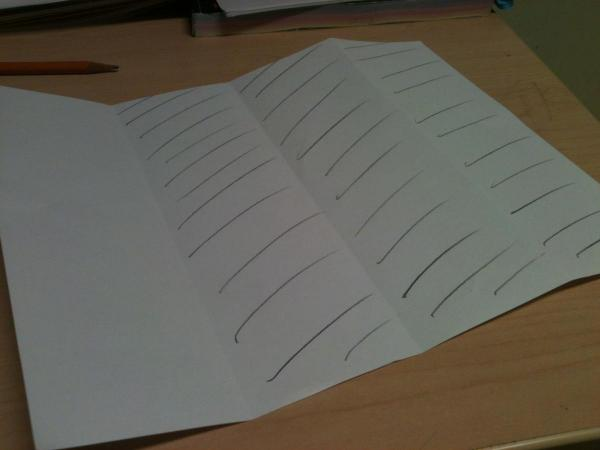First, form the fraction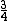by folding the paper into quarters and shading three of them in.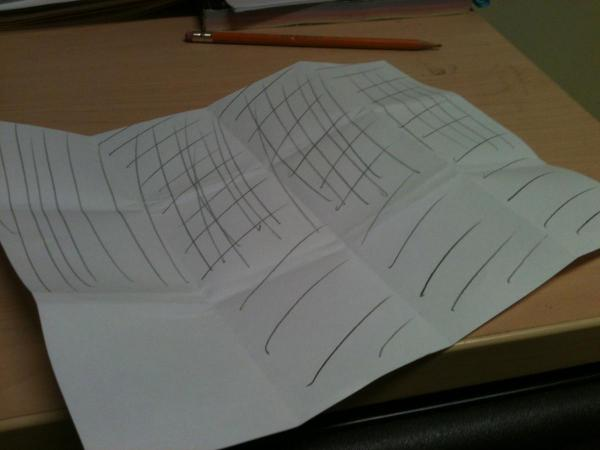Now fold the paper in the other direction into thirds, and shade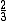, ideally in the other direction. Where your two shadings have overlapped is the product of your two fractions, in this case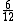.

Symmetry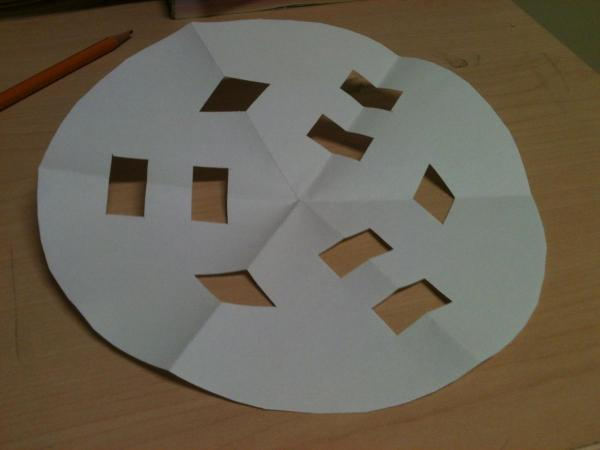Here is an example of folding the paper around the centre to produce rotational symmetry. I worked with a student to produce snowflakes with  9 points, 12 points, and other points, after watching this interesting video by Vi Hart

Tessellations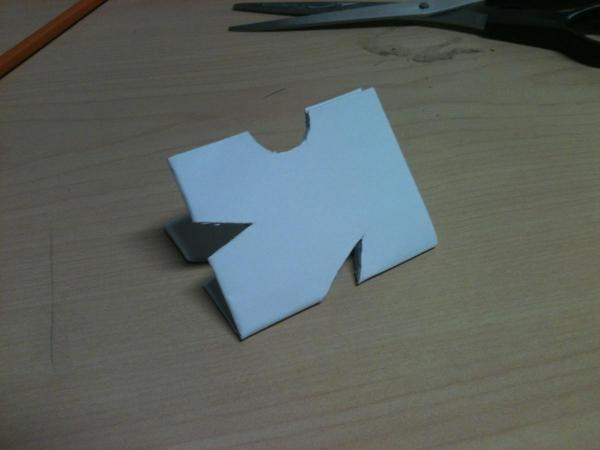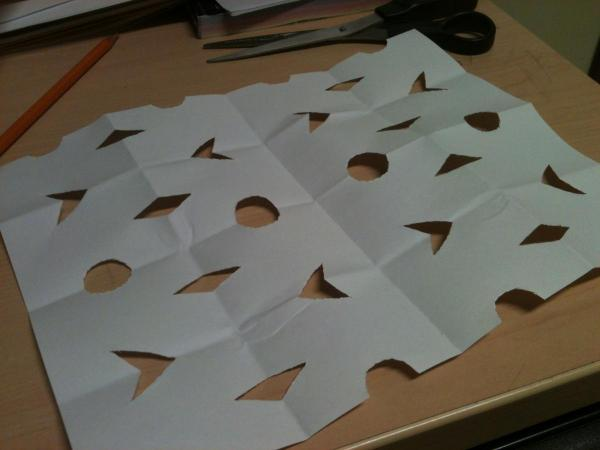If you fold a paper in half a bunch of times, you can create a tesselation by cutting portions of the paper out. The number of folds and the size of the repeated portion of the tessellation have an interesting relationship.

Circle geometry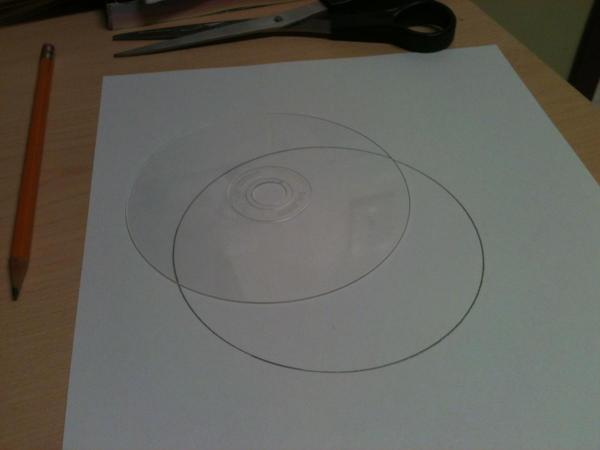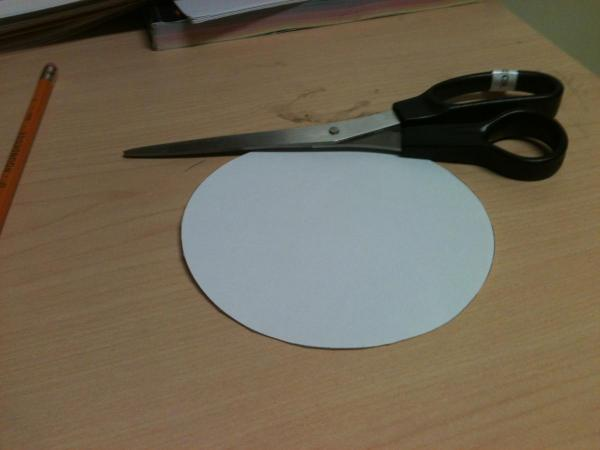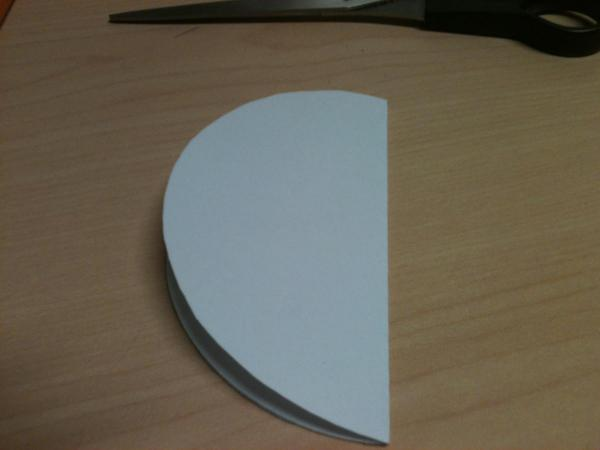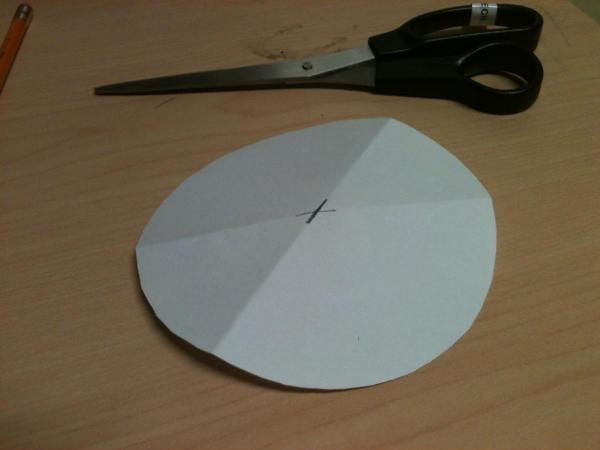If you very carefully cut a circle out of a piece of paper (which will finally give you a use for all of those CDs you have laying around you aren’t using anymore), you can prove quite a large number of the theorems from circle geometry by folding the paper in certain ways.

For example, if you fold the paper in half twice in two different directions, the intersection of the folds has a useful property.

For further resources on paper folding and mathematics, see this TED talk by Robert Lang, this book on the mathematics of paper-folding, and this useful PDF describing some geometry theorems that can be demonstrated through paper folding. See also this very interesting article on fraction flags (via @DwyerTeacher).